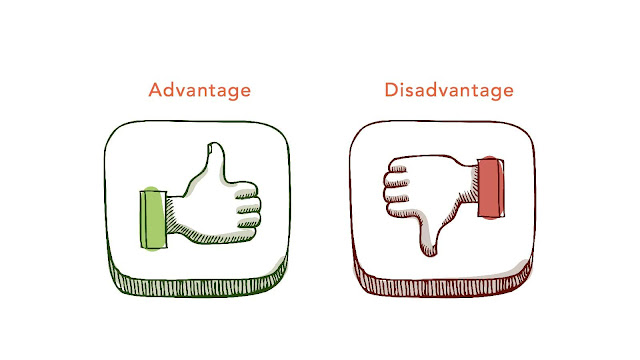# HSSlive: Plus One & Plus Two Notes & Solutions for Kerala State Board

## Monday, March 13, 2023Advantages And Disadvantages Of 2D Rectangular Matrix In C

## What is 2D Rectangular Matrix In C?

In C programming language, a 2D rectangular matrix can be represented as a multidimensional array. Here is an example of how to declare, initialize, and access elements in a 2D rectangular matrix in C:

`#include <stdio.h> int main() { // declare and initialize a 3x4 matrix int matrix = {{1, 2, 3, 4}, {5, 6, 7, 8}, {9, 10, 11, 12}}; // access an element in the matrix int element = matrix; // element at row 1, column 2 (7) // print the matrix for(int i=0; i<3; i++) { for(int j=0; j<4; j++) { printf("%d ", matrix[i][j]); } printf("\n"); } return 0; } `

In this example, the matrix is declared as a 2D array with 3 rows and 4 columns. The elements are initialized using nested braces. The element at row 1 and column 2 is accessed using the indices . The matrix is printed using two nested for-loops to iterate over the rows and columns.

Note that in C, a 2D rectangular matrix is stored in memory as a contiguous block of elements, with each row stored one after the other. This means that accessing elements in row-major order (i.e., iterating over the rows first) can be more efficient than accessing elements in column-major order.

## Advantages of 2D Rectangular Matrix In C

Here are some advantages of using a 2D rectangular matrix in C programming language:

1. Easy to declare: A 2D rectangular matrix can be easily declared as a multidimensional array in C. The syntax is straightforward and can be easily understood.

2. Efficient access to elements: Accessing elements in a 2D rectangular matrix is efficient in C because the memory is contiguous. This allows for quick and easy access to individual elements using their row and column indices.

3. Good for math operations: C programming language is known for its strong support for math operations. 2D rectangular matrices are used extensively in mathematical computations, and C provides a wide range of built-in functions and libraries for working with matrices.

4. Useful for representing images: 2D rectangular matrices can be used to represent images in C. Each element in the matrix can represent a pixel value, and the matrix can be easily manipulated to perform operations such as resizing, rotation, and flipping.

5. Compact representation of data: A 2D rectangular matrix is a compact representation of data in C. This makes it easy to store and manipulate large amounts of data in memory.

Overall, a 2D rectangular matrix is a powerful tool for working with data in C. Its efficiency, ease of use, and strong support for mathematical operations make it a popular choice for a wide range of applications.

## Disadvantages of 2D Rectangular Matrix In C

Here are some disadvantages of using a 2D rectangular matrix in C programming language:

1. Fixed size: A 2D rectangular matrix in C has a fixed size, which means that it cannot be resized dynamically. This can be problematic if the size of the data changes frequently or if the size is unknown in advance.

2. Inefficient for sparse data: If a significant portion of the data is missing or empty, a 2D rectangular matrix can be inefficient in C because it requires memory space to be allocated for all the elements, including the empty ones.

3. Memory overhead: A 2D rectangular matrix in C requires additional memory overhead to store the row and column indices of each element. This overhead can be a concern for large matrices and may increase memory usage.

4. Complexity: Performing complex operations on 2D rectangular matrices in C can be computationally expensive and time-consuming, especially for large matrices. This can impact the performance of the program.

5. Difficulty in passing to functions: Passing a 2D rectangular matrix to a function in C can be complicated because it requires the function to know the dimensions of the matrix in advance. This can lead to code duplication and a lack of flexibility.

Overall, while 2D rectangular matrices are a useful data structure in C programming language, they are not always the most efficient or appropriate data structure for every use case. It is important to consider the specific requirements of the application and the potential trade-offs before using a 2D rectangular matrix.

Share: